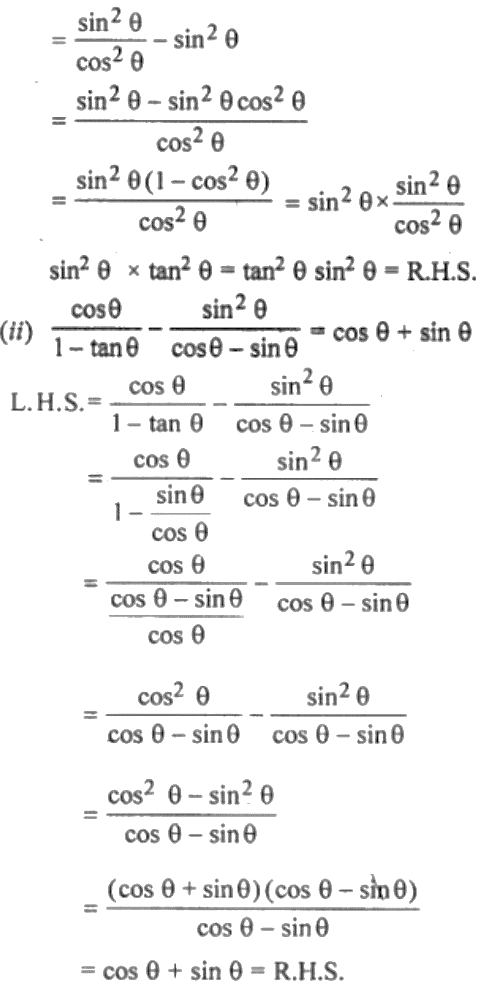Guru

# (i) tan2 θ – sin2 θ = tan2 θ sin2 θ (ii) cos θ/ (1 – tan θ) – sin2 θ/ (cos θ – sin θ) = cos θ + sin θ

• 0

This is an important question taken from the Book Book- ML Aggarwal
Board- ICSE
Publication- Avichal
Chapter- Trigonometric Identities
Chapter number-18
We have to prove that the given equation holds true.

(i) tan2 θ – sin2 θ = tan2 θ sin2 θ

(ii) cos θ/ (1 – tan θ) – sin2 θ/ (cos θ – sin θ) = cos θ + sin θ

Class 10, chapter 18, trigonometric identities, ques no. 19

Share